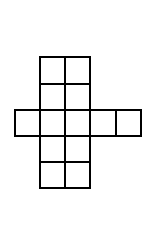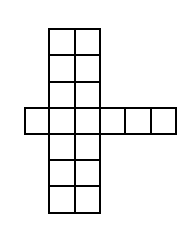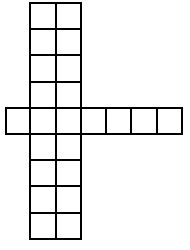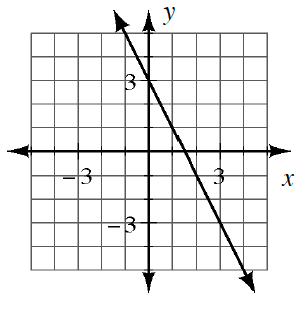### Home > CCA > Chapter 2 > Lesson 2.2.2 > Problem2-67

2-67.

Each part (a) through (d) below represents a different tile pattern. For each one, determine how the pattern is growing and the number of tiles in Figure 0. Homework Help ✎

1.Figure 2 Figure 3 Figure 4
• How many more squares does Figure 3 have than Figure 2?

1.• Make a slope triangle between two points on the graph to find the slope, or the growth rate.

Growth rate: $−2$

1. $y=3x−14$

Number of tiles in Figure 0: $−14$

1.  $x$ $−3$ $−2$ $−1$ $0$ $1$ $2$ $3$ $y$ $18$ $13$ $8$ $3$ $−2$ $−7$ $−12$

What is the difference between each $y$-value?
What is the value of $y$ when $x$ is $0$?